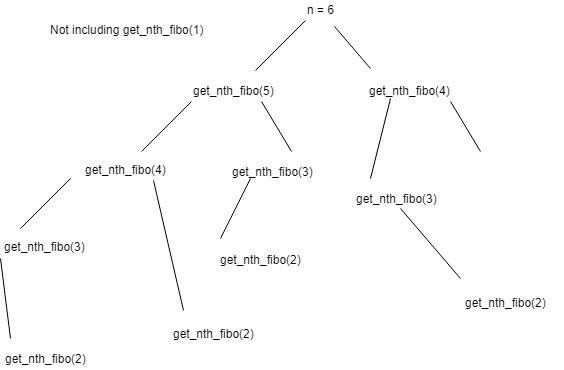# Question:

The Fibonacci sequence is defined as follows: the first number of the sequence is 0, the second number is 1, and the nth number is the sum of the (n – 1)th and (n – 2)th numbers. Write a function that takes in an integer n and returns the nth Fibonacci number.

A Fibonacci series is a series of numbers in which each number ( Fibonacci number ) is the sum of the two preceding numbers. The simplest is the series 0,1, 1, 2, 3, 5, 8, etc.

```Example: Sample input: 6 Sample output: 5 ```

```(0, 1, 1, 2, 3, 5) ```

# Solution:

1. Recursion

Recursion is the process which comes into existence when a function calls a copy of itself to work on a smaller problem. For any recursive function we must first find the base case that will exit the recursion. If the base case is not hit, then the function can go into a infinite loop and we might run into a stack overflow error.

From the above given definition of Fibonacci numbers we can say that

1st number = 0
2nd number = 1
3rd number = 1
4th number = 2
5th number =3
6th number = 5

Hence the base casses can be –
For n == 1 return 0
for n == 2 return 1
Let us put this into code

So, for n == 3

get_nth_fibo(2) + get_nth_fibo(1)
1 + 0 = 1

Let us try that again for `n==6`Adding up all the get_nth_fibo(2) in the above diagram you will get – 5, which is the correct answer for this `n==6.`

2. With caching

As you can see from the above diagram, we calculate certain values more than once, thus increasing the time complexity of our code. For n==6 get_nth_fibo(3) is calculated 3 times. We can avoid that by using a cache or a key value pair of already calculated values of n.

### Sliding Sidebar